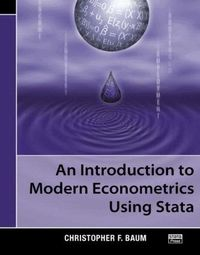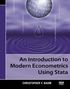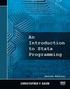Fler böcker inom
Format
Häftad (Paperback / softback)
Språk
Engelska
Antal sidor
341
Utgivningsdatum
2006-08-01
Förlag
Stata Press
Illustratör/Fotograf
Illustrations
Illustrationer
Illustrations
Dimensioner
240 x 180 x 10 mm
Vikt
720 g
Antal komponenter
1
ISBN
9781597180139

Du kanske gillarAn Introduction to Modern Econometrics Using Stata

1063
Skickas inom 2-5 vardagar.
Fri frakt inom Sverige för privatpersoner.
Integrating a contemporary approach to econometrics with the powerful computational tools offered by Stata, An Introduction to Modern Econometrics Using Stata focuses on the role of method-of-moments estimators, hypothesis testing, and specification analysis and provides practical examples that show how the theories are applied to real data sets using Stata. As an expert in Stata, the author successfully guides readers from the basic elements of Stata to the core econometric topics. He first describes the fundamental components needed to effectively use Stata. The book then covers the multiple linear regression model, linear and nonlinear Wald tests, constrained least-squares estimation, Lagrange multiplier tests, and hypothesis testing of nonnested models. Subsequent chapters center on the consequences of failures of the linear regression model's assumptions. The book also examines indicator variables, interaction effects, weak instruments, underidentification, and generalized method-of-moments estimation. The final chapters introduce panel-data analysis and discrete- and limited-dependent variables and the two appendices discuss how to import data into Stata and Stata programming. Presenting many of the econometric theories used in modern empirical research, this introduction illustrates how to apply these concepts using Stata. The book serves both as a supplementary text for undergraduate and graduate students and as a clear guide for economists and financial analysts.

Passar bra ihop

1.2. +
3.De som köpt den här boken har ofta också köpt An Introduction to Stata Programming, Second Ed... av Christopher F Baum (häftad).

Kundrecensioner

Har du läst boken? Sätt ditt betyg »

Innehållsförteckning

PREFACE NOTATION AND TYPOGRAPHY INTRODUCTION An Overview of Stata's Distinctive Features Installing the Necessary Software Installing the Support Materials WORKING WITH ECONOMIC AND FINANCIAL DATA IN STATA The Basics Common Data Transformations ORGANIZING AND HANDLING ECONOMIC DATA Cross-Sectional Data and Identifier Variables Time-Series Data Pooled Cross-Sectional Time-Series Data Panel Data Tools for Manipulating Panel Data Combining Cross-Sectional and Time-Series Datasets Creating Long-Format Datasets with Append The Reshape Command Using Stata for Reproducible Research LINEAR REGRESSION Introduction Computing Linear Regression Estimates Interpreting Regression Estimates Presenting Regression Estimates Hypothesis Tests, Linear Restrictions, and Constrained Least Squares Computing Residuals and Predicted Values Computing Marginal Effects Appendix A: Regression as a Least-Squares Estimator Appendix B: The Large-Sample VCE for Linear Regression SPECIFYING THE FUNCTIONAL FORM Introduction Specification Error Endogeneity and Measurement Error REGRESSION WITH NON-I.I.D. ERRORS The Generalized Linear Regression Model Heteroskedasticity in the Error Distribution Serial Correlation in the Error Distribution REGRESSION WITH INDICATOR VARIABLES Testing for Significance of a Qualitative Factor Regression with Qualitative and Quantitative Factors Seasonal Adjustment with Indicator Variables Testing for Structural Stability and Structural Change INSTRUMENTAL-VARIABLES ESTIMATORS Introduction Endogeneity in Economic Relationships 2SLS The ivreg Command Identification and Tests of Overidentifying Restrictions Computing IV Estimates ivreg2 and GMM Estimation Testing and Overidentifying Restrictions in GMM Testing for Heteroskedasticity in the IV Context Testing the Relevance of Instruments Durbin-Wu-Hausman Tests for Endogeneity in IV Estimation Appendix A: Omitted-Variables Bias Appendix B: Measurement Error PANEL-DATA MODELS FE and RE Models IV Models for Panel Data Dynamic Panel-Data Models Seemingly Unrelated Regression Models Moving-Window Regression Estimates MODELS OF DISCRETE AND LIMITED DEPENDENT VARIABLES Binomial Logit and Probit Models Ordered Logit and Probit Models Truncated Regression and Tobit Models Incidental Truncation and Sample-Selection Models Bivariate Probit and Probit with Selection APPENDIX A: GETTING THE DATA INTO STATA Inputting Data from ASCII Text Files and Spreadsheets Importing Data from Other Package Formats APPENDIX B: THE BASICS OF STATA PROGRAMMING Local and Global Macros Scalars Loop Constructs Matrices return and ereturn The Program and Syntax Statements Using Mata Functions in Stata Programs REFERENCES AUTHOR INDEX SUBJECT INDEX